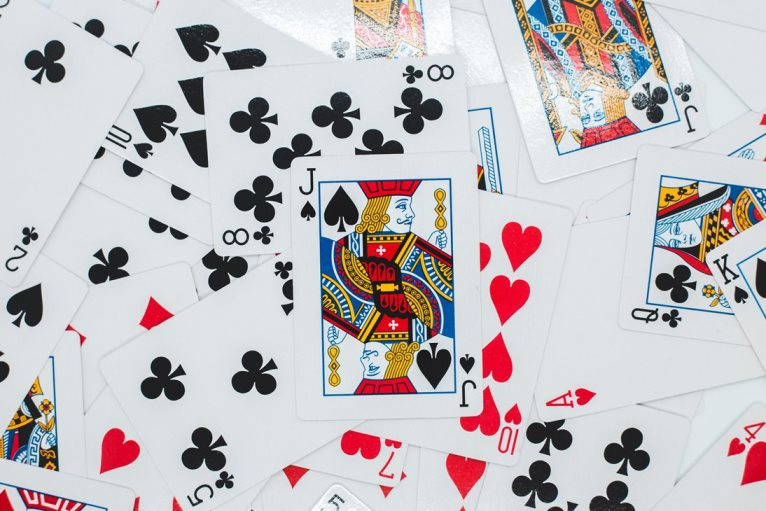# Mutually Exclusive Events – Understanding Mutually Exclusive Events in Probability with Examples

Probability, at its core, serves as a measure of the likelihood of an event’s occurrence. But when dealing with multiple events, how do they relate to one another? This brings us to the topic of “mutually exclusive events”.In this Blog Post we will learn:

## 1. What are Mutually Exclusive Events?

Mutually exclusive events are events that cannot happen at the same time. In other words, the occurrence of one event excludes the occurrence of the other. If two events are mutually exclusive, the probability of them both occurring simultaneously is zero.

Mathematically, if $A$ and $B$ are two mutually exclusive events, then:

$P(A \cap B) = 0$

Where:
– $P(A \cap B)$ denotes the probability of both $A$ and $B$ occurring at the same time.

## 2. Real-Life Examples

To grasp this concept more intuitively, let’s explore a few examples:

Example 1: Consider the act of flipping a fair coin. It can either land on heads (H) or tails (T), but not both at the same time. So, the events “landing on heads” and “landing on tails” are mutually exclusive.

Example 2: Think about rolling a fair six-sided die. The outcomes are 1, 2, 3, 4, 5, or 6. The event “rolling a 3” and the event “rolling a 4” are mutually exclusive because you can’t roll both numbers in a single throw.

Example 3: Consider choosing a card from a standard deck of 52 cards. If you pick one card, the events “drawing an ace” and “drawing a queen” are mutually exclusive. You can’t draw both an ace and a queen in a single pick.

## 3. Mutually Exclusive vs. Independent Events

It’s vital not to confuse “mutually exclusive” with “independent” events. Two events are independent if the occurrence of one event does not affect the probability of the other event happening. Mutually exclusive events are always dependent because if one occurs, the other cannot.

For instance, in our coin flip example:
– Landing on heads and landing on tails are mutually exclusive (they can’t both happen).
– However, the result of one coin flip doesn’t affect the result of a subsequent flip. Therefore, the events are independent across separate trials.

## 4. Calculating the Probability of Mutually Exclusive Events

For two mutually exclusive events $A$ and $B$, the probability that either $A$ or $B$ will occur is the sum of their individual probabilities:

$P(A \cup B) = P(A) + P(B)$

Example: In a standard deck of 52 cards, what’s the probability of drawing either a king or a queen?

• Probability of drawing a king, $P(King)$ = 4/52
• Probability of drawing a queen, $P(Queen)$ = 4/52

Using our formula:
$P(King \cup Queen) = \frac{4}{52} + \frac{4}{52} = \frac{8}{52} = \frac{1}{6.5}$

Thus, the probability of drawing either a king or a queen is 1/6.5 or approximately 0.1538 (15.38%).

## 5. Conclusion

Understanding the concept of mutually exclusive events is fundamental in probability theory and its applications. Whether you’re analyzing a card game, interpreting statistical data, or solving complex business problems, recognizing events that cannot occur simultaneously can aid in deriving accurate conclusions.

## Similar Articles

### Logistic Regression – A Complete Tutorial With Examples in RCourse Preview

## Machine Learning A-Z™: Hands-On Python & R In Data Science

### Free Sample Videos:#### Machine Learning A-Z™: Hands-On Python & R In Data Science#### Machine Learning A-Z™: Hands-On Python & R In Data Science#### Machine Learning A-Z™: Hands-On Python & R In Data Science#### Machine Learning A-Z™: Hands-On Python & R In Data Science